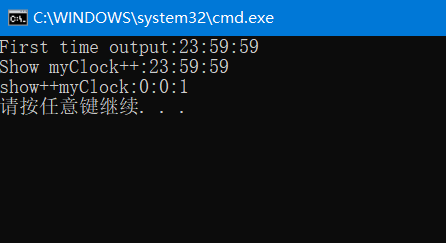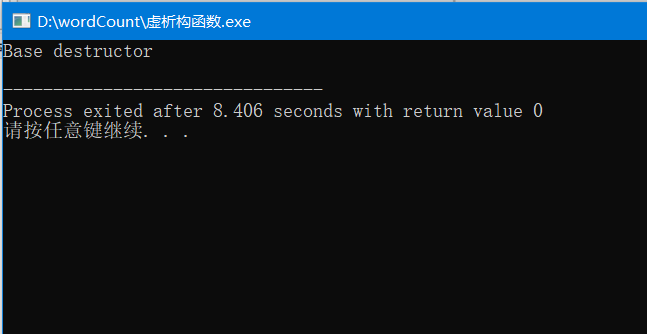# 重载与多态

### 重载

#### 运算符重载

{

}

#include<iostream>
using namespace std;
class Clock{
public:
Clock(int hour = 0, int minute = 0, int second = 0);
void showTime()const;
Clock& operator++();
Clock operator++(int);
private:
int hour, minute, second;
};

Clock::Clock(int hour, int minute, int second){
if (0 <= hour&&hour < 24 && 0 <= minute&&minute < 60 && 0 <= second&&second < 60){
this->hour = hour;
this->minute = minute;
this->second = second;

}
else
cout << "Time error!" << endl;
}

void Clock::showTime()const{
cout << hour << ":" << minute << ":" << second << endl;
}
Clock&Clock::operator++(){
second++;
if (second >= 60){
second -= 60;
minute++;
if (minute >= 60){
minute -= 60;
hour = (hour + 1) % 24;
}
}
return*this;
}
Clock Clock::operator++(int){
Clock old = *this;
++(*this);
return old;
}

int main(){
Clock myClock(23, 59, 59);
cout << "First time output:";
myClock.showTime();
cout << "Show myClock++:";
(myClock++).showTime();
cout << "show++myClock:";
(++myClock).showTime();
return 0;
}#### 虚函数

virtual 函数类型 函数名(形参表);


virtual ~类名();


#include<iostream>
using namespace std;
class Base
{
public:
~Base();

};
Base::~Base()
{
cout<<"Base destructor"<<endl;

}
class Derived:public Base {
public:
Derived();
~Derived();
private:
int *p;

};
Derived::Derived(){
p=new int(0);

}
Derived::~Derived(){
cout<<"Derievd destructor"<<endl;
delete p;
}
void fun(Base*b){
delete b;
}
int main()
{
Base*b=new Derived();
fun(b);
return0;
}### 纯虚函数与抽象类

virtual 函数类型 函数名(参数表)=0;


posted @ 2019-10-27 22:46  znlovewxl  阅读(555)  评论(0编辑  收藏  举报